Ask your WordPress questions! Pay money and get answers fast!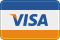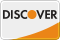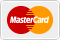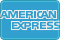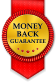# Formidable Form Auto Populate Multiple Rows in Repeater Field. WordPress

• SOLVED

So here what I need:
1. In Step 2 of the form I have repeater field. Here a user can add row as many as he wants.

2. So, from step lets say a user added 3 rows.

3. I have another repeater field in step 7. So, if in step two a user adeed 3 rows I need to auto populate 3 rows in step 7 with 1 Field values from step 2.

Here is working relevant document how you can auto populate rows in repeating section:
https://formidableforms.com/knowledgebase/frm_setup_new_fields_vars/#kb-auto-populate-multiple-rows-in-a-repeater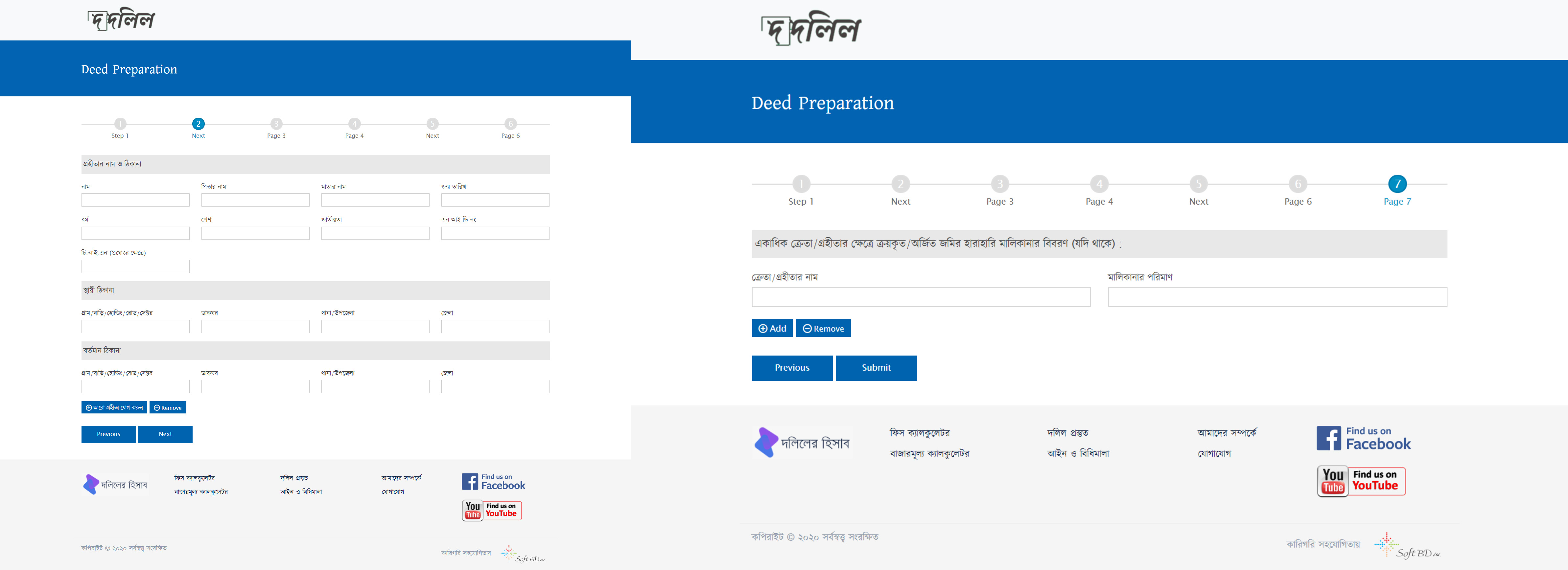2020-04-16

Hello Jihan,
I hope you are in a good health, Could you send me your login info to my email to do this task
[email protected]

I have emailed you the wordpress login and ftp. Plz let me know if you any question. Thanks.

Thanks for info,
Although that and also I can't understand the Bengali language.
but you will use this code and it will work.

</code>
function frm_auto_populate_repeating_fields( \$values, \$field ) {
if ( \$field->id == 147 ) {//Replace with the ID of your field
// if ( ! isset( \$_POST['item_meta'][ \$field->id ] ) ) {
\$first_field = 149;
\$second_field = 150;

\$values[ 'value' ] = array(
'form' => '24',
'row_ids' => array( 0, 1, 2,3 ),
0 => array( 0 => '', \$first_field => \$_POST['item_meta'], \$second_field =>\$_POST['item_meta'] ),
1 => array( 0 => '', \$first_field => \$_POST['item_meta'], \$second_field => \$_POST['item_meta'] ),
3 => array( 0 => '', \$first_field => \$_POST['item_meta'], \$second_field => \$_POST['item_meta'] ),
);
}
//}
return \$values;
}
</code>

I have tried the code.
1. Its auto populating row but not correctly.
2. Only First row has value in step 7.
3. Also We need to make it dynamic so a user might add 2 row or 3 row or 4 row. So it should be co-related with with step 2. If in Step 2 a user add 2 row in step 7 we get 2 row. If a user add 3 row in Step 2 then in Step 7 we get 3 row.

Thanks.

I have updated this ```0 => array( 0 => '', \$first_field => \$_POST['item_meta'], \$second_field => \$_POST['item_meta'] ), 1 => array( 0 => '', \$first_field => \$_POST['item_meta'], \$second_field => \$_POST['item_meta'] ), 3 => array( 0 => '', \$first_field => \$_POST['item_meta'], \$second_field => \$_POST['item_meta'] ),```

It's now auto populating field values properly.

The only thing left: We need to make it dynamic so a user might add 2 row or 3 row or 4 row. So it should be co-related with with step 2. If in Step 2 a user add 2 row in step 7 we get 2 row. If a user add 3 row in Step 2 then in Step 7 we get 3 row.

Hi Jihan,

No problem, try this code and it will do what you want exactly

``` add_filter('frm_setup_new_fields_vars', 'frm_auto_populate_repeating_fields', 20, 2); function frm_auto_populate_repeating_fields( \$values, \$field ) { if ( \$field->id == 147 ) { // if ( ! isset( \$_POST['item_meta'][ \$field->id ] ) ) { \$first_field = 149; \$second_field = 150; \$count = count(\$_POST['item_meta']['row_ids']); \$rows = \$_POST['item_meta']['row_ids']; \$values[ 'value' ] = array( 'form' => '24', 'row_ids' => array(implode(",",\$rows)) ); for (\$x = 0; \$x <= \$count; \$x++){ \$values[ 'value' ][\$x] = array( \$x => '', \$first_field => \$_POST['item_meta'][\$x], \$second_field => \$_POST['item_meta'][\$x] ); } } //} return \$values; } ```

1. Working fine but creating 1 empty rows each time. I guess these problem arise as because there is 1 row always. And we are creating equal number of rows from step 2 so there us +1 always.

``` add_filter('frm_setup_new_fields_vars', 'frm_auto_populate_repeating_fields', 20, 2); function frm_auto_populate_repeating_fields( \$values, \$field ) { if ( \$field->id == 147 ) { // if ( ! isset( \$_POST['item_meta'][ \$field->id ] ) ) { \$first_field = 149; \$second_field = 150; \$count = count(\$_POST['item_meta']['row_ids']); \$rows = \$_POST['item_meta']['row_ids']; \$values[ 'value' ] = array( 'form' => '24', 'row_ids' => array(implode(",",\$rows)) ); for (\$x = 0; \$x < \$count; \$x++){ \$values[ 'value' ][\$x] = array( \$x => '', \$first_field => \$_POST['item_meta'][\$x], \$second_field => \$_POST['item_meta'][\$x] ); } } //} return \$values; } ```

Great. Works Fine.

There are few errors:
Warning: count(): Parameter must be an array or an object that implements Countable in /home/dolil131/public_html/dolil.com/calculator/wp-content/themes/dolil-preparation/functions.php on line 222

Warning: implode(): Invalid arguments passed in /home/dolil131/public_html/dolil.com/calculator/wp-content/themes/dolil-preparation/functions.php on line 227

How do I suppress them?

``` add_filter('frm_setup_new_fields_vars', 'frm_auto_populate_repeating_fields', 20, 2); function frm_auto_populate_repeating_fields( \$values, \$field ) { if ( \$field->id == 147 ) { //if ( ! isset( \$_POST['item_meta'][ \$field->id ] ) ) { \$first_field = 149; \$second_field = 150; if (\$_POST['item_meta']){ \$count = count(\$_POST['item_meta']['row_ids']); } if ( \$_POST['item_meta']){ is_array( \$_POST['item_meta']['row_ids']) ? \$rows = \$_POST['item_meta']['row_ids'] : \$rows=array(); } else{ \$rows = array(); } \$values[ 'value' ] = array( 'form' => '24', 'row_ids' => array(implode(",",\$rows)) ); for (\$x = 0; \$x < \$count; \$x++){ \$values[ 'value' ][\$x] = array( \$x => '', \$first_field => \$_POST['item_meta'][\$x], \$second_field => \$_POST['item_meta'][\$x] ); } } //} return \$values; } ```